﻿ Project Maths | Tutorials for Geometry
Working together to improve teaching & learning Leagan Gaeilge
Professional Development ›› Tutorials for GeometryWelcome to our brand new tutorials for Geometry. Each tutorial will take you through a construction/theorem from the Geometry Course of Study at Junior or Leaving Certificate.The tutorials will explore both conceptual and procedural knowledge  for teaching and learning . Each video concludes with a list of additional resources available on the website for use in the classroom.

### Constructions in Geometry

Bisector of an Angle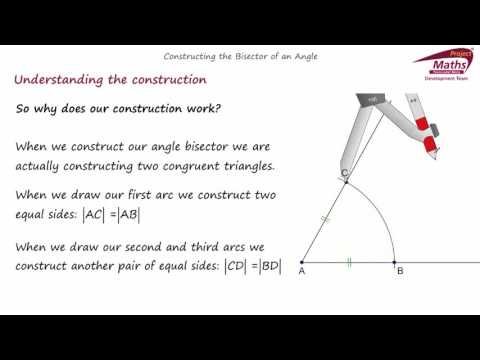How do we construct the bisector of an angle? How does the construction work? How ca...

Perpendicular Bisector of a Line Segment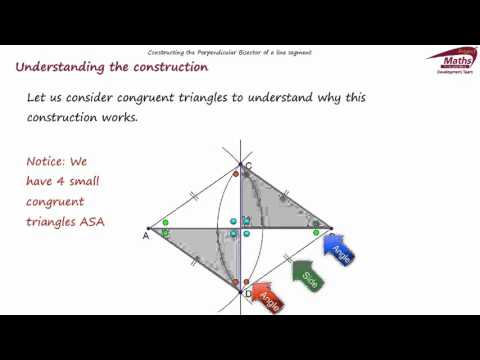How do we construct the perpendicular bisector of a line segment? How does the const...

Circumcentre and Circumcircle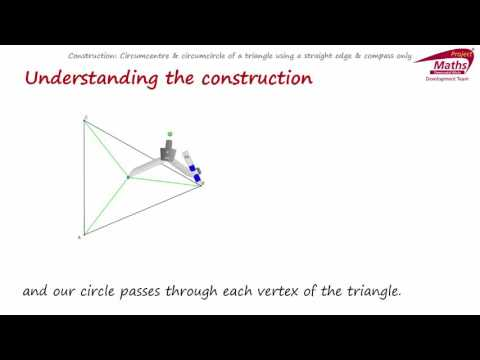How do we construct the circumcircle of a triangle? How does the construction work? ...

Incentre and Incircle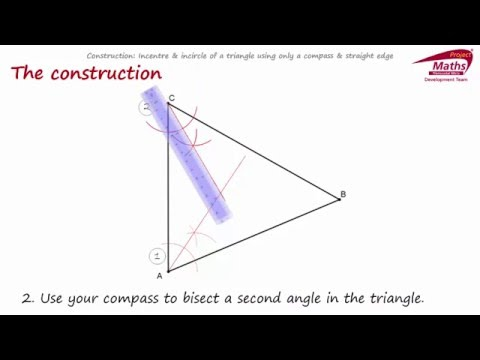How do we construct the incircle of a triangle? How does the construction work? How ...

Angle of 60 degrees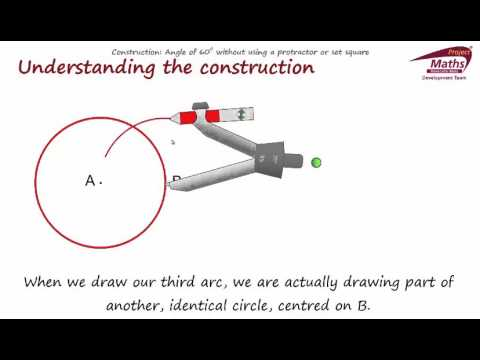How do we construct the an angle of 60 degrees without a protractor or set square? H...

### Theorems in Geometry

Vertically-opposite angles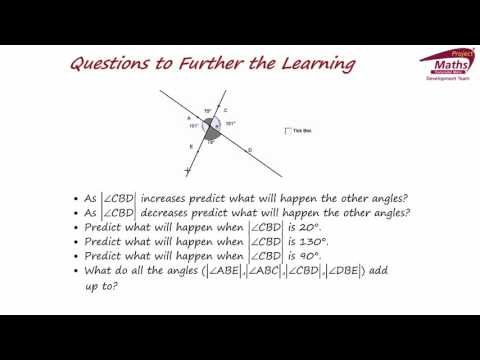Teaching a theorem using discovery learning, algebra as sense making and generalisin...